Updating search results...

# 51 Results

View
Selected filters:
• CCSS.Math.Practice.MP.5Only Sharing Permitted
CC BY-NC-ND
Rating
4.0 stars

This lesson unit is intended to help teachers assess how well students are able to classify solutions to a pair of linear equations by considering their graphical representations. In particular, this unit aims to help teachers identify and assist students who have difficulties in: using substitution to complete a table of values for a linear equation; identifying a linear equation from a given table of values; and graphing and solving linear equations.

Subject:
Algebra
Material Type:
Assessment
Lesson Plan
Provider:
Shell Center for Mathematical Education
Provider Set:
Mathematics Assessment Project (MAP)
04/26/2013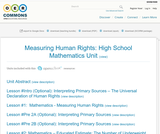Unrestricted Use
CC BY
Rating
5.0 stars

In this unit, students will read and interpret primary sources to address the question “How do we measure the attainment of human rights?” By exploring the Universal Declaration of Human Rights, the UN’s Guide to Indicators of Human Rights, and data about development indicators from multiple databases, students will unpack the complexities of using indicators to measure human rights.

Subject:
Mathematics
Material Type:
Assessment
Lesson Plan
Author:
Tamar Posner
01/28/2016Conditional Remix & Share Permitted
CC BY-NC-SA
Rating
3.25 stars

This task was developed by high school and postsecondary mathematics and design/pre-construction educators, and validated by content experts in the Common Core State Standards in mathematics and the National Career Clusters Knowledge & Skills Statements. It was developed with the purpose of demonstrating how the Common Core and CTE Knowledge & Skills Statements can be integrated into classroom learning - and to provide classroom teachers with a truly authentic task for either mathematics or CTE courses.

Subject:
Architecture and Design
Geometry
Ratios and Proportions
Material Type:
Activity/Lab
Assessment
Homework/Assignment
Lesson Plan
Provider:
National Association of State Directors of Career Technical Education Consortium
Provider Set:
Career Technical Education
03/05/2012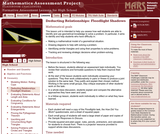Only Sharing Permitted
CC BY-NC-ND
Rating
0.0 stars

This lesson unit is intended to help teachers assess how well students are able to identify and use geometrical knowledge to solve a problem. In particular, this unit aims to identify and help students who have difficulty in: making a mathematical model of a geometrical situation; drawing diagrams to help with solving a problem; identifying similar triangles and using their properties to solve problems; and tracking and reviewing strategic decisions when problem-solving.

Subject:
Geometry
Material Type:
Assessment
Lesson Plan
Provider:
Shell Center for Mathematical Education
Provider Set:
Mathematics Assessment Project (MAP)
04/26/2013Only Sharing Permitted
CC BY-NC-ND
Rating
4.5 stars

This lesson unit is intended to help assess how well students are able to interpret and use scale drawings to plan a garden layout. This involves using proportional reasoning and metric units.

Subject:
Algebra
Geometry
Ratios and Proportions
Material Type:
Assessment
Lesson Plan
Provider:
Shell Center for Mathematical Education
Provider Set:
Mathematics Assessment Project (MAP)
04/26/2013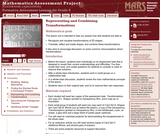Only Sharing Permitted
CC BY-NC-ND
Rating
4.0 stars

This lesson unit is intended to help teachers assess how well students are able to: recognize and visualize transformations of 2D shapes; and translate, reflect and rotate shapes, and combine these transformations. It also aims to encourage discussion on some common misconceptions about transformations.

Subject:
Geometry
Material Type:
Assessment
Lesson Plan
Provider:
Shell Center for Mathematical Education
Provider Set:
Mathematics Assessment Project (MAP)
04/26/2013Only Sharing Permitted
CC BY-NC-ND
Rating
4.5 stars

This lesson unit is intended to help teachers assess how well students are able to visualize two-dimensional cross-sections of representations of three-dimensional objects. In particular, the lesson will help you identify and help students who have difficulties recognizing and drawing two-dimensional cross-sections at different points along a plane of a representation of a three-dimensional object.

Subject:
Geometry
Material Type:
Assessment
Lesson Plan
Provider:
Shell Center for Mathematical Education
Provider Set:
Mathematics Assessment Project (MAP)
04/26/2013Only Sharing Permitted
CC BY-NC-ND
Rating
0.0 stars

This lesson unit is intended to help teachers assess how well students are able to: work with concepts of congruency and similarity, including identifying corresponding sides and corresponding angles within and between triangles; Identify and understand the significance of a counter-example; Prove, and evaluate proofs in a geometric context.

Subject:
Geometry
Material Type:
Assessment
Lesson Plan
Provider:
Shell Center for Mathematical Education
Provider Set:
Mathematics Assessment Project (MAP)
04/26/2013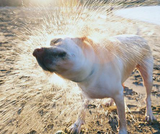Rating
0.0 stars

Rational Numbers

Type of Unit: Concept

Prior Knowledge

Students should be able to:

Solve problems with positive rational numbers.
Plot positive rational numbers on a number line.
Understand the equal sign.
Use the greater than and less than symbols with positive numbers (not variables) and understand their relative positions on a number line.
Recognize the first quadrant of the coordinate plane.

Lesson Flow

The first part of this unit builds on the prerequisite skills needed to develop the concept of negative numbers, the opposites of numbers, and absolute value. The unit starts with a real-world application that uses negative numbers so that students understand the need for them. The unit then introduces the idea of the opposite of a number and its absolute value and compares the difference in the definitions. The number line and positions of numbers on the number line is at the heart of the unit, including comparing positions with less than or greater than symbols.

The second part of the unit deals with the coordinate plane and extends student knowledge to all four quadrants. Students graph geometric figures on the coordinate plane and do initial calculations of distances that are a straight line. Students conclude the unit by investigating the reflections of figures across the x- and y-axes on the coordinate plane.

Subject:
Mathematics
Numbers and Operations
Material Type:
Unit of Study
Provider:
Pearson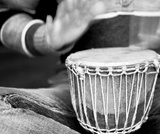Rating
0.0 stars

Getting Started

Type of Unit: Introduction

Prior Knowledge

Students should be able to:

Understand ratio concepts and use ratios.
Use ratio and rate reasoning to solve real-world problems.
Identify and use the multiplication property of equality.

Lesson Flow

This unit introduces students to the routines that build a successful classroom math community, and it introduces the basic features of the digital course that students will use throughout the year.

An introductory card sort activity matches students with their partner for the week. Then over the course of the week, students learn about the routines of Opening, Work Time, Ways of Thinking, Apply the Learning (some lessons), Summary of the Math, Reflection, and Exercises. Students learn how to present their work to the class, the importance of students’ taking responsibility for their own learning, and how to effectively participate in the classroom math community.

Students then work on Gallery problems, to further explore the resources and tools and to learn how to organize their work.

The mathematical work of the unit focuses on ratios and rates, including card sort activities in which students identify equivalent ratios and match different representations of an equivalent ratio. Students use the multiplication property of equality to justify solutions to real-world ratio problems.

Subject:
Mathematics
Material Type:
Unit of Study
Provider:
Pearson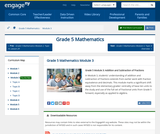Conditional Remix & Share Permitted
CC BY-NC-SA
Rating
4.0 stars

In Module 3, students' understanding of addition and subtraction of fractions extends from earlier work with fraction equivalence and decimals. This module marks a significant shift away from the elementary grades' centrality of base ten units to the study and use of the full set of fractional units from Grade 5 forward, especially as applied to algebra.

Subject:
Ratios and Proportions
Material Type:
Module
Provider:
New York State Education Department
Provider Set:
EngageNY
11/27/2012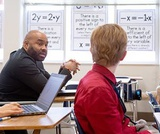Unrestricted Use
CC BY
Rating
5.0 stars

Mathematically Productive Instructional Routines (MPIR) are short (10ish minutes), daily exercises aimed at building number sense. These six different MPIR are part of the Mathematically Productive Instructional Routines collection from the Washington Office of Public Instruction and the Washington Association of Educational Service Districts.

Subject:
Mathematics
Material Type:
Teaching/Learning Strategy
Author:
Barbara Soots
Washington OSPI Mathematics Department
Washington OSPI OER Project
04/05/2021Only Sharing Permitted
CC BY-NC-ND
Rating
4.0 stars

This lesson unit is intended to help you assess how well students are able to: Perform arithmetic operations, including those involving whole-number exponents, recognizing and applying the conventional order of operations; Write and evaluate numerical expressions from diagrammatic representations and be able to identify equivalent expressions; apply the distributive and commutative properties appropriately; and use the method for finding areas of compound rectangles.

Subject:
Geometry
Material Type:
Assessment
Lesson Plan
Provider:
Shell Center for Mathematical Education
Provider Set:
Mathematics Assessment Project (MAP)
04/26/2013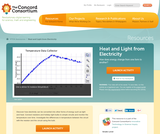Rating
0.0 stars

Discover how electricity can be converted into other forms of energy such as light and heat. Connect resistors and holiday light bulbs to simple circuits and monitor the temperature over time. Investigate the differences in temperature between the circuit with the resistor and the circuit using the bulb.

Subject:
Education
Chemistry
Physics
Material Type:
Activity/Lab
Diagram/Illustration
Provider:
Concord Consortium
Provider Set:
Concord Consortium Collection
Author:
The Concord Consortium
12/12/2011Unrestricted Use
CC BY
Rating
0.0 stars

The COVID-19 Pandemic is a clear example of how science and society are connected. This unit explores how different communities are differentially impacted by the virus through the lens of historical inequities in society. In the context of decisions their families make, students explore the basics of how the virus affects people, and design investigations to explore how it spreads from person to person, and what we can do to prevent that spread.

Subject:
Life Science
Material Type:
Lesson
Lesson Plan
Unit of Study
Provider:
OpenSciEd
Author:
OpenSciEd
08/02/2021Rating
0.0 stars

Elementary grade students investigate heat transfer in this activity to design and build a solar oven, then test its effectiveness using a temperature sensor. It blends the hands-on activity with digital graphing tools that allow kids to easily plot and share their data. Included in the package are illustrated procedures and extension activities. Note Requirements: This lesson requires a "VernierGo" temperature sensing device, available for ~ \$40. This item is part of the Concord Consortium, a nonprofit research and development organization dedicated to transforming education through technology. The Consortium develops digital learning innovations for science, mathematics, and engineering.

Subject:
Engineering
Education
Life Science
Ecology
Forestry and Agriculture
Mathematics
Chemistry
Physics
Material Type:
Activity/Lab
Diagram/Illustration
Lecture Notes
Provider:
Concord Consortium
Provider Set:
Concord Consortium Collection
Author:
The Concord Consortium
04/02/2013Conditional Remix & Share Permitted
CC BY-NC
Rating
0.0 stars

Subject:
Mathematics
Material Type:
Full Course
Provider:
Pearson
10/06/2016Rating
0.0 stars

Getting Started

Type of Unit: Introduction

Prior Knowledge

Students should be able to:

Solve and write numerical equations for whole number addition, subtraction, multiplication, and division problems.
Use parentheses to evaluate numerical expressions.
Identify and use the properties of operations.

Lesson Flow

In this unit, students are introduced to the rituals and routines that build a successful classroom math community and they are introduced to the basic features of the digital course that they will use throughout the year.

An introductory card sort activity matches students with their partner for the week. Then over the course of the week, students learn about the lesson routines: Opening, Work Time, Ways of Thinking, Apply the Learning, Summary of the Math, and Reflection. Students learn how to present their work to the class, the importance of taking responsibility for their own learning, and how to effectively participate in the classroom math community.

Students then work on Gallery problems to further explore the program’s technology resources and tools and learn how to organize their work.

The mathematical work of the unit focuses on numerical expressions, including card sort activities in which students identify equivalent expressions and match an expression card to a word card that describes its meaning. Students use the properties of operations to identify equivalent expressions and to find unknown values in equations.

Subject:
Mathematics
Material Type:
Unit of Study
Provider:
PearsonRating
0.0 stars

Fractions and Decimals

Type of Unit: Concept

Prior Knowledge

Students should be able to:

Multiply and divide whole numbers and decimals.
Multiply a fraction by a whole number.
Multiply a fraction by another fraction.
Write fractions in equivalent forms, including converting between improper fractions and mixed numbers.
Understand the meaning and structure of decimal numbers.

Lesson Flow

This unit extends students’ learning from Grade 5 about operations with fractions and decimals.

The first lesson informally introduces the idea of dividing a fraction by a fraction. Students are challenged to figure out how many times a 14-cup measuring cup must be filled to measure the ingredients in a recipe. Students use a variety of methods, including adding 14 repeatedly until the sum is the desired amount, and drawing a model. In Lesson 2, students focus on dividing a fraction by a whole number. They make a model of the fraction—an area model, bar model, number line, or some other model—and then divide the model into whole numbers of groups. Students also work without a model by looking at the inverse relationship between division and multiplication. Students explore methods for dividing a whole number by a fraction in Lesson 3, for dividing a fraction by a unit fraction in Lesson 4, and for dividing a fraction by another fraction in Lesson 6. Students examine several methods and models for solving such problems, and use models to solve similar problems.

Students apply their learning to real-world contexts in Lesson 6 as they solve word problems that require dividing and multiplying mixed numbers. Lesson 7 is a Gallery lesson in which students choose from a number of problems that reinforce their learning from the previous lessons.

Students review the standard long-division algorithm for dividing whole numbers in Lesson 8. They discuss the different ways that an answer to a whole number division problem can be expressed (as a whole number plus a remainder, as a mixed number, or as a decimal). Students then solve a series of real-world problems that require the same whole number division operation, but have different answers because of how the remainder is interpreted.

Students focus on decimal operations in Lessons 9 and 10. In Lesson 9, they review addition, subtraction, multiplication, and division with decimals. They solve decimal problems using mental math, and then work on a card sort activity in which they must match problems with diagram and solution cards. In Lesson 10, students review the algorithms for the four basic decimal operations, and use estimation or other methods to place the decimal points in products and quotients. They solve multistep word problems involving decimal operations.

In Lesson 11, students explore whether multiplication always results in a greater number and whether division always results in a smaller number. They work on a Self Check problem in which they apply what they have learned to a real-world problem. Students consolidate their learning in Lesson 12 by critiquing and improving their work on the Self Check problem from the previous lesson. The unit ends with a second set of Gallery problems that students complete over two lessons.

Subject:
Mathematics
Ratios and Proportions
Material Type:
Unit of Study
Provider:
PearsonRating
0.0 stars

Expressions

Type of Unit: Concept

Prior Knowledge

Students should be able to:

Write and evaluate simple expressions that record calculations with numbers.
Use parentheses, brackets, or braces in numerical expressions and evaluate expressions with these symbols.
Interpret numerical expressions without evaluating them.

Lesson Flow

Students learn to write and evaluate numerical expressions involving the four basic arithmetic operations and whole-number exponents. In specific contexts, they create and interpret numerical expressions and evaluate them. Then students move on to algebraic expressions, in which letters stand for numbers. In specific contexts, students simplify algebraic expressions and evaluate them for given values of the variables. Students learn about and use the vocabulary of algebraic expressions. Then they identify equivalent expressions and apply properties of operations, such as the distributive property, to generate equivalent expressions. Finally, students use geometric models to explore greatest common factors and least common multiples.

Subject:
Mathematics
Algebra
Material Type:
Unit of Study
Provider:
Pearson